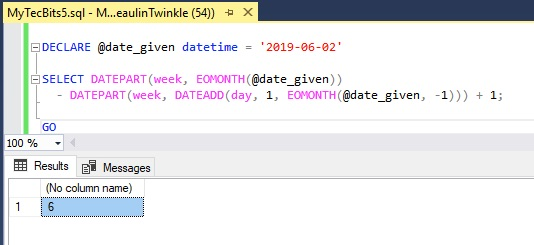# How To Find Number Of Weeks In A Month in SQL Server?

Here is a couple of methods to find the number of weeks in a month including the partial weeks considering different start day of week.

## 1. Considering Start Day Of Week Is From System (Eg. Sunday)

For this method, I will be using the technique I have used before to find the first day of the month and last day of the month from the given date. Then, by slightly modifying the technique to find the week number in a month to find the total number of weeks in the month. Here is the script:

```DECLARE @date_given datetime = '2019-06-02'

SELECT DATEPART(week, EOMONTH(@date_given))
- DATEPART(week, DATEADD(day, 1, EOMONTH(@date_given, -1))) + 1;

GO
```

## 2. Considering Start Day Of Week Is First Day Of Month

This method is straight forward. We will consider the first day of week is same as the first day of the month. For example if June of 2019 the start of month (2019/06/01) is Saturday. Then consider the week start day is Saturday. In that case you can use the below query to get the number of weeks in a month based on a given day.

```DECLARE @date_given datetime = '2019-06-02'

SELECT (DATEPART(dd, EOMONTH(@date_given)) / 7) +
CASE WHEN (DATEPART(dd, EOMONTH(@date_given)) % 7) > 0 THEN 1 ELSE 0 END;

GO
```

## Reference

### Related Articles & Tools

This site uses Akismet to reduce spam. Learn how your comment data is processed.